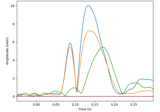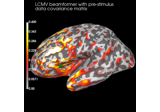# mne.cov.regularize#

mne.cov.regularize(cov, info, mag=0.1, grad=0.1, eeg=0.1, exclude='bads', proj=True, seeg=0.1, ecog=0.1, hbo=0.1, hbr=0.1, fnirs_cw_amplitude=0.1, fnirs_fd_ac_amplitude=0.1, fnirs_fd_phase=0.1, fnirs_od=0.1, csd=0.1, dbs=0.1, rank=None, scalings=None, verbose=None)[source]#

Regularize noise covariance matrix.

This method works by adding a constant to the diagonal for each channel type separately. Special care is taken to keep the rank of the data constant.

Note

This function is kept for reasons of backward-compatibility. Please consider explicitly using the `method` parameter in `mne.compute_covariance()` to directly combine estimation with regularization in a data-driven fashion. See the FAQ for more information.

Parameters:
cov`Covariance`

The noise covariance matrix.

info`mne.Info`

The `mne.Info` object with information about the sensors and methods of measurement. (Used to get channel types and bad channels).

mag`float` (default 0.1)

Regularization factor for MEG magnetometers.

grad`float` (default 0.1)

Regularization factor for MEG gradiometers. Must be the same as `mag` if data have been processed with SSS.

eeg`float` (default 0.1)

Regularization factor for EEG.

exclude`list` | ‘bads’ (default ‘bads’)

projbool (default `True`)

Apply projections to keep rank of data.

seeg`float` (default 0.1)

Regularization factor for sEEG signals.

ecog`float` (default 0.1)

Regularization factor for ECoG signals.

hbo`float` (default 0.1)

Regularization factor for HBO signals.

hbr`float` (default 0.1)

Regularization factor for HBR signals.

fnirs_cw_amplitude`float` (default 0.1)

Regularization factor for fNIRS CW raw signals.

fnirs_fd_ac_amplitude`float` (default 0.1)

Regularization factor for fNIRS FD AC raw signals.

fnirs_fd_phase`float` (default 0.1)

Regularization factor for fNIRS raw phase signals.

fnirs_od`float` (default 0.1)

Regularization factor for fNIRS optical density signals.

csd`float` (default 0.1)

Regularization factor for EEG-CSD signals.

dbs`float` (default 0.1)

Regularization factor for DBS signals.

rank`None` | ‘info’ | ‘full’ | `dict`

This controls the rank computation that can be read from the measurement info or estimated from the data. When a noise covariance is used for whitening, this should reflect the rank of that covariance, otherwise amplification of noise components can occur in whitening (e.g., often during source localization).

`None`

The rank will be estimated from the data after proper scaling of different channel types.

`'info'`

The rank is inferred from `info`. If data have been processed with Maxwell filtering, the Maxwell filtering header is used. Otherwise, the channel counts themselves are used. In both cases, the number of projectors is subtracted from the (effective) number of channels in the data. For example, if Maxwell filtering reduces the rank to 68, with two projectors the returned value will be 66.

`'full'`

The rank is assumed to be full, i.e. equal to the number of good channels. If a `Covariance` is passed, this can make sense if it has been (possibly improperly) regularized without taking into account the true data rank.

`dict`

Calculate the rank only for a subset of channel types, and explicitly specify the rank for the remaining channel types. This can be extremely useful if you already know the rank of (part of) your data, for instance in case you have calculated it earlier.

This parameter must be a dictionary whose keys correspond to channel types in the data (e.g. `'meg'`, `'mag'`, `'grad'`, `'eeg'`), and whose values are integers representing the respective ranks. For example, `{'mag': 90, 'eeg': 45}` will assume a rank of `90` and `45` for magnetometer data and EEG data, respectively.

The ranks for all channel types present in the data, but not specified in the dictionary will be estimated empirically. That is, if you passed a dataset containing magnetometer, gradiometer, and EEG data together with the dictionary from the previous example, only the gradiometer rank would be determined, while the specified magnetometer and EEG ranks would be taken for granted.

The default is `None`.

New in v0.17.

New in v0.18: Support for ‘info’ mode.

scalings

Data will be rescaled before rank estimation to improve accuracy. See `mne.compute_covariance()`.

New in v0.17.

verbose

Control verbosity of the logging output. If `None`, use the default verbosity level. See the logging documentation and `mne.verbose()` for details. Should only be passed as a keyword argument.

Returns:
reg_cov`Covariance`

The regularized covariance matrix.

## Examples using `mne.cov.regularize`#Compute a sparse inverse solution using the Gamma-MAP empirical Bayesian method

Compute a sparse inverse solution using the Gamma-MAP empirical Bayesian methodCompute cross-talk functions for LCMV beamformers

Compute cross-talk functions for LCMV beamformers